# SSAT Elementary Level Math : How to find the whole from the part

## Example Questions

### Example Question #71 : How To Find The Whole From The Part

Which line is longer?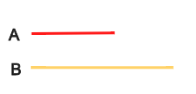Line A

Line B

They are the same length

Line B

Explanation:

Line B is longer than line A.

### Example Question #72 : How To Find The Whole From The Part

Which line is longer?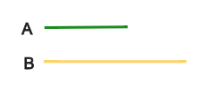Line A

They are the same length

Line B

Line B

Explanation:

Line B is longer than line A.

### Example Question #73 : How To Find The Whole From The Part

Which line is longer?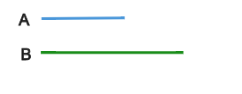Line B

Line A

They are the same length

Line B

Explanation:

Line B is longer than line A.

### Example Question #74 : How To Find The Whole From The Part

Which shape is smaller?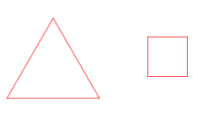They are the same size

The triangle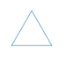The square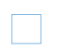The squareExplanation:

The square is smaller than the triangle.

### Example Question #75 : How To Find The Whole From The Part

Which shape is smaller?They are the same size

The circle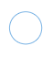The squareThe circleExplanation:

The circle is smaller than the square.

### Example Question #76 : How To Find The Whole From The Part

Which shape is smaller?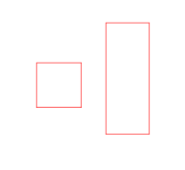The rectangleThey are the same size

The squareThe squareExplanation:

The square is smaller than the rectangle.

### Example Question #77 : How To Find The Whole From The Part

Which shape is smaller?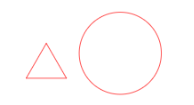The triangleThe circleThey are the same size

The triangleExplanation:

The triangle is smaller than the circle.

### Example Question #78 : How To Find The Whole From The Part

Which shape is smaller?The rectangleThey are the same size

The circleThe circleExplanation:

The circle is smaller than the rectangle.

### Example Question #79 : How To Find The Whole From The Part

Which shape is smaller?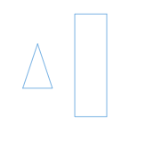The rectangleThey are the same size

The triangle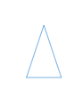The triangleExplanation:

The triangle is smaller than the rectangle.

### Example Question #80 : How To Find The Whole From The Part

Which shape is smaller?The circleThe rectangleThey are the same size